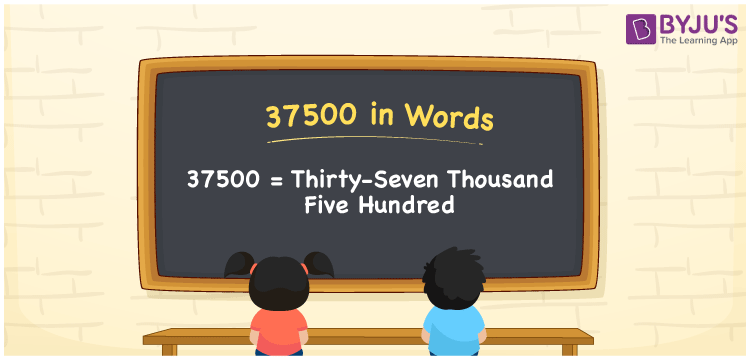# 37500 in Words

We can write 37500 in words as Thirty-seven thousand Five hundred. Suppose a magazine contains 37500 words of content in it; we can say that this magazine has Thirty-seven thousand Five hundred words. In this article, you will learn how to write the cardinal number 37500 in word form using a place value chart.

 37500 in words Thirty-seven thousand Five hundred Thirty-seven thousand Five hundred in Numbers 37500

## 37500 in English words

Generally, we can write numbers in words using the English alphabet. So, we can read 37500 in English as “Thirty-seven thousand Five hundred”.## How to Write 37500 in Words?

In this section, you will understand the method of writing the number 37500 in words using a five-column place value chart since it has 5 digits. This can be shown as:

 Ten thousands Thousands Hundreds Tens Ones 3 7 5 0 0

From the above table, we can expand the digits according to their place values as:

3 × Ten thousand + 7 × Thousand + 5 × Hundred + 0 × Ten + 0 × One

= 3 × 10000 + 7 × 1000 + 5 × 100 + 0 × 10 + 0 × 1

= 30000 + 7000 + 500

= Thirty thousand + Seven thousand + Five hundred

= Thirty-seven thousand Five hundred

Therefore, 37500 in words = Thirty-seven thousand Five hundred.

### Facts About the Number 37500

37500 is a natural number that is the successor of 37499 and the predecessor of 37501.

37500 in words – Thirty-seven thousand Five hundred

Is 37500 an odd number? – No

Is 37500 an even number? – Yes

Is 37500 a perfect square number? – No

Is 37500 a perfect cube number? – No

Is 37500 a prime number? – No

Is 37500 a composite number? – Yes

## Frequently Asked Questions on 37500 in Words

Q1

### How do you write 37500 in English words?

We can express 37500 in English words as Thirty-seven thousand Five hundred. If this number conveys the money or the price of something, we can say that Thirty-seven thousand Five hundred rupees.
Q2

### How do you write 37500 on a cheque?

On a cheque, 37500 can be written as Thirty-seven thousand Five hundred rupees only and we generally mention the amount as Rs. 37500/-.
Q3

### Express the value of 22000 + 15500 in words.

22000 + 15500 = 37500 The value 22000 + 15500 is 37500, i.e. Thirty-seven thousand Five hundred.